## Özkan Öcalan: An improved oscillation criterion for first order difference equations, p.65-73

### Abstract:

This paper is concerned with the oscillatory behavior of first order difference equation with general argument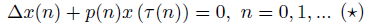where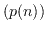is a sequence of nonnegative real numbers and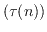is a sequence of integers. Let the number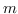be defined by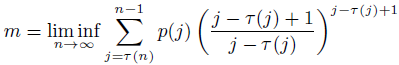. It is proved that, all solutions of Equation (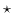) oscillate if the condition m>1 is satisfied.

Key Words: Delay difference equation, general argument, oscillation.

2000 Mathematics Subject Classification: Primary: 39A10;
Secondary: 39A21.